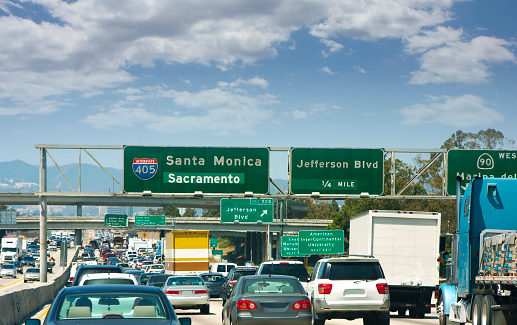## A Traffic Flow Problem

Consider you are driving in peak LA traffic, and notice the adjacent highway lane has a volume of 5,600 vehicles/hr, with a 15 km/hr average vehicle speed. What is the time spacing between vehicles (center to center) in seconds?Hint
The average vehicle speed is not necessary to solve this problem and is simply there to throw you off.
Hint 2
Convert from hours to seconds.
The average vehicle speed is not necessary to solve this problem and is simply there to throw you off. Focusing on the given volume, we can say that in 1 hour, 5600 vehicles pass by. More specifically, it can be fractionally expressed as:
$$\frac{1\:hour}{5600\:vehicles}$$$Since we are solving for vehicle spacing, we can do some unit conversions to get an answer. $$spacing=\frac{1\:hour}{5600\:vehicles}\cdot \frac{60\:min}{1\:hour}\cdot \frac{60\:sec}{1\:min}$$$
$$=\frac{3600\:sec}{5600\:vehicles}=0.64\:sec/vehicle$$\$
0.64 sec/vehicle